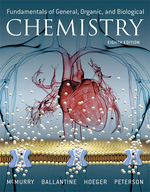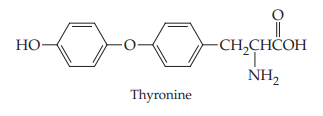×
Get Full Access to Fundamentals Of General, Organic, And Biological Chemistry (Mastering Chemistry) - 8 Edition - Chapter 14 - Problem 14.61
Get Full Access to Fundamentals Of General, Organic, And Biological Chemistry (Mastering Chemistry) - 8 Edition - Chapter 14 - Problem 14.61

×ISBN: 9780134015187 2044

## Solution for problem 14.61 Chapter 14

Fundamentals of General, Organic, and Biological Chemistry (Mastering Chemistry) | 8th Edition

• Textbook Solutions
• 2901 Step-by-step solutions solved by professors and subject experts
• Get 24/7 help from StudySoup virtual teaching assistantsFundamentals of General, Organic, and Biological Chemistry (Mastering Chemistry) | 8th Edition

4 5 1 315 Reviews
31
5
Problem 14.61

Thyroxine (Section 14.9) is synthesized in the body by reaction of thyronine with iodine. Write the reaction, and tell what kind of process is occurring (Hint: See Section 13.5).Step-by-Step Solution:
Step 1 of 3

Math 2311 Written Homework 2 (Sections 1.5 & 2.1) Name:___Giang Tran________________________ PeopleSoft ID:_1372369___________ Instructions:  Homework will NOT be accepted through email or in person. Homework must be submitted through CourseWare BEFORE the deadline.  Print out this file and complete the problems.  Use blue or black ink or a dark pencil.  Write your solutions in the space provided. You must show all work for full credit.  Submit this assignment at http://www.casa.uh.edu under "Assignments" and choose whw2. 1. Section 1.5, Problem 2 a. - The distribution of data is skewed left because the mean is smaller than the median (mean=74.71 < median= 76.00) - Possible outliers: IQR= Q3 – Q1= 84 – 68 = 16 1.5IQR = 24 => Outlier boundaries: [Q1 – 1.5IQR, Q3 +1.5IQR] = [68 – 24, 84 -24]= [44,108] => This data has an outlier, which is 35 - The middle 50% lies between 68 and 84, which locates at the median 76 - Range = Max – Min = 94 – 35 = 59 b. Construct boxplot 2. Using the data called SAT in the mosaic Data package, create boxplots for both the math and verbal score variables. Write one to two sentences comparing those scores.  For math score variables: > fivenum(~math,data=SAT)  443.0 474.0 497.5 540.0 592.0 > bwplot(~math,data=SAT)  For verbal score variables: > fivenum(~verbal,data=SAT)  401 427 448 491 516 > bwplot(~verbal,data=SAT) => Both math and verbal scores’ distributions are skewed right The math scores are generally higher than verbal scores And the math scores have a wider range (thus more variable) than the verbal scores 3. Section 2.1, Problem 12 > choose(8,4)  70 8C 4 = 70 There are 70 ways to form a committee of 4 from a group of 8 people 4. Section 2.1, Problem 18 > choose(8,2)  28 > choose(6,2)  15 > choose(8,2)*choose(6,2)  420 To draw 2 white marbles from 8 white marbles, we8C2 = 28 ways To draw 2 black marbles from 6 black marbles, w6Ch2 = 15 ways  To draw the 4 marbles so that 2 will be white and 2 will be black, we have 8C 2 x 6C 2 = 28 x 15 = 420 ways 5. Section 2.1, Problem 22 > factorial(4)  24 > factorial(6)  720 > factorial(4)*factorial(6)  17280 To arrange the tallest 4 in the back, we have 4! = 24 ways To arrange the rest of people, we have (10­4)! = 6! = 720 ways  So, for a group of 10 people to line up for a picture so that the tallest 4 stand in the back, we have 4! x (10 – 4)! = 24 x 720 = 17280 (ways) Math 2311 Written Homework 4 (Sections 3.1­3.3) Name:___Giang Tran___________________________ PeopleSoft ID:_1372369________ Instructions:  Homework will NOT be accepted through email or in person. Homework must be submitted through CourseWare BEFORE the deadline.  Print out this file and complete the problems.  Use blue or black ink or a dark pencil.  Write your solutions in the space provided. You must show all work for full credit.  Submit this assignment at http://www.casa.uh.edu under "Assignments" and choose whw4. 1. Section 3.1, Problem 4 Mean of X: > 1*(.15)+2*(.2)+3*(0.1)+4*(.2)+5*(.1)+6*(.15)+7*(.1)  3.75 So, mean of X is 3.75 2. Section 3.1, Problem 10 Portfolio A: Var (A) = E(A ) ­ E(A)2 = > (-1500*-1500*.2+-100*-100*.1+500*500*.4+1500*1500*0.2+3500*3500*0.1)-(-1500*.2+- 100*.1+500*.4+1500*0.2+3500*0.1)*(-1500*.2+-100*.1+500*.4+1500*0.2+3500*0.1) = 1934400  Standard deviation of Portfolio A = square root of Var(A) = 1390.83 Portfolio B: 2 2 Var (B) = E(B ) ­ E(B) = > (-2500*-2500*.2+-500*-500*.1+1500*1500*.3+2500*2500*.3+3500*3500*.1)-(-2500*.2+- 500*.1+1500*.3+2500*.3+3500*.1)*(-2500*.2+-500*.1+1500*.3+2500*.3+3500*.1) = 4050000  Standard deviation of Portfolio B = square root of Var(B) = 2012.46 So, these standard deviations contradicts answer for problem 9, which says it is better to choose Portfolio B (since “Standard deviation of Portfolio B” is greater than “Standard deviation of Portfolio A”, so Portfolio B have more variations from the mean than Portfolio A) 3. Section 3.1, Problem 20 Mean (X) -1*.3+0*.1+1*.5+2*.1 = 0.4 Variance (X= > (-1*-1*.3+0*0*.1+1*1*.5+2*2*.1)-(-1*.3+0*.1+1*.5+2*.1)*(-1*.3+0*.1+1*.5+2*.1)= 1.04 Standard deviation (X) = square root of Variance (X) = 1.02 So, we have: Mean (W) = Mean (3+2X) = 2 Mean (X) +3 = 2*.4 + 3= 3.8 Variance (W) = Variance (3+2X) = 2 Variance (X) = 4.16 Standard deviation (W) = square root of Variance (W) = 2.04 4. Section 3.2, Problem 10 a. Proportion of groups will exactly four of the six households have a DVD player P(X=4) = > dbinom(4,6,.9)= 0.098415 b. Proportion of groups will at most two of the households have a DVD player P(X<=2) = > pbinom(2,6,.9) = 0.00127 5. Section 3.2, Problem 14 a. Probability that exactly 2 have never been married P(X=2) = > dbinom(2,10,.2)= 0.3019899 b. Probability that at most 2 have never been married P(X<=2) = > pbinom(2,10,.2)= 0.6777995 c. Probability that at least 8 have been married = Probability that at most 2 have never been married = 0.6777995 6. Section 3.2, Problem 16 a. The probability that exactly five complete the program P(X=5) = dbinom(5,7,.7) = 0.3176523 b. The probability that five or more complete the program P(X>=5) = 1 – P(X<5) = 1 – P(X<=4) = 1-pbinom(4,7,.7) = 0.6470695 c. The average number of people out of the seven that went to the training that will complete the program E(X) = np = 7*.7 = 4.9 ~ 5 people 7. Section 3.3, Problem 8 a. The probability that the quarterback throws 3 incomplete passes before he has a completion P(X=4)= dgeom(3,.44) = 0.07727104 b. The number of passes can the quarterback expect to throw before he completes a pass E(X)= 1/p= 1/.44= 2.272727 c. The probability that it takes more than 5 attempts before he completes a pass P(X>5)= 1­ P(X<=5) = 1-pgeom(4,.44)= 0.05507318 d. The probability that he attempts more than 7 passes before he completes one P(X>7)= 1­ P(X<=7) = 1-pgeom(6,.44)= 0.01727095 8. Section 3.3, Problem 10 a. The probability that she sells more than 20 policies P(X>20) = 1 – P(X<=20) = 1-pbinom(20,120,.15)= 0.2556828 b. The expected number of policies she sells E(X) = np = 120*.15 = 18

Step 2 of 3

Step 3 of 3

##### ISBN: 9780134015187

The full step-by-step solution to problem: 14.61 from chapter: 14 was answered by Aimee Notetaker, our top Chemistry solution expert on 04/25/22, 03:57PM. Since the solution to 14.61 from 14 chapter was answered, more than 200 students have viewed the full step-by-step answer. The answer to “?Thyroxine (Section 14.9) is synthesized in the body by reaction of thyronine with iodine. Write the reaction, and tell what kind of process is occurring (Hint: See Section 13.5).” is broken down into a number of easy to follow steps, and 29 words. Fundamentals of General, Organic, and Biological Chemistry (Mastering Chemistry) was written by Aimee Notetaker and is associated to the ISBN: 9780134015187. This full solution covers the following key subjects: . This expansive textbook survival guide covers 281 chapters, and 456 solutions. This textbook survival guide was created for the textbook: Fundamentals of General, Organic, and Biological Chemistry (Mastering Chemistry), edition: 8.

## Discover and learn what students are asking

Statistics: Informed Decisions Using Data : Data Collection
?In Problems 1–5, provide a definition using your own words. 4. Observational study

Statistics: Informed Decisions Using Data : Scatter Diagrams and Correlation
?True or False: Correlation implies causation.

Unlock Textbook Solution